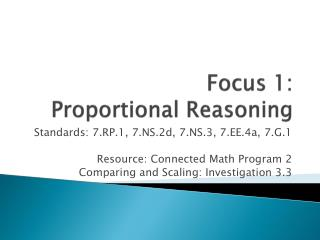DownloadDownload PresentationFocus 1: Proportional Reasoning

# Focus 1: Proportional Reasoning

Télécharger la présentation## Focus 1: Proportional Reasoning

- - - - - - - - - - - - - - - - - - - - - - - - - - - E N D - - - - - - - - - - - - - - - - - - - - - - - - - - -
##### Presentation Transcript

1. Focus 1: Proportional Reasoning Standards: 7.RP.1, 7.NS.2d, 7.NS.3, 7.EE.4a, 7.G.1 Resource: Connected Math Program 2 Comparing and Scaling: Investigation 3.3

2. Comparing and Scaling Ratio, Proportion, and Percent Investigation #3- CMP2

3. Mathematical & Problem-SolvingLearning Goals for Comparing & Scaling Rates • Examine & Connect the idea of unit rate to what you already know about ratios and about linear relationships (3.1) • Further develop understanding of unit rates and how to compute and interpret them (3.2) • Work with the important application of rates to miles per hour (speed) (3.2) • Introduce the concept of “average” or “steady” rate of progress (3.2) • Introduce and formalize the meaning of unit rate and computation strategies for computing unit rates (3.3) • Relate unit rate to the slope of the line representing the equation of the underlying relationship (3.3) • Confront the issue of what it means to divide in rate situations

4. Investigation 3.3Learning Target • Introduce and formalize the meaning of unit rate and computation strategies for computing unit rates • Relate unit rate to the slope of the line representing the equation of the underlying relationship

5. Getting Ready for Problem 3.3 The ads below use rates to describe sale prices. To compare prices in sales such as these, it’s often useful to find a unit rate. A unit rate is a rate in which one of the numbers being compared is 1 unit. The comparisons “45 miles per gallon,” “\$3.50 per hour,” “8.5 kilometers per hour,” and “two sandwiches for each person” are all unit rates. “Per gallon” means “for one gallon” and “per hour” means “for one hour.”

6. 3.3 Comparing CD Prices MUSIC CITY CD Sale! 5 for \$49.95 CD WORLD Huge Savings! 7 for \$65.80

7. 3.3 Unit Rates and Equations Use unit rates to compare the ad prices and to find the costs of various numbers of CDs at each store. • Which store has the lower price per CD? • For each store, write an equation (a rule) that you can use to calculate the cost c for any purchase of n compact discs. • Use the equations you just wrote for Question B. Write new equations to include 5% sales tax on any purchase.

8. Suppose a Web site sells CDs for \$8.99 per disc. There is no tax, but there is a shipping charge of \$5 for any order. Write an equation to give the cost c of any order for n discs from the Web site. • Use your equation from Question C or make a rate table to answer each question. • How many discs do you have to order from the Web site to get a better deal than buying from Music City? • How many discs do you have to order from the Web site to get a better deal than buying from CD World?

9. Explore 3.3Have you thought about? • What are the variables in the situation, and what letters are good representations for the variables? • Which seems to be the independent variable, and which the dependent variable?

10. Class Discussion & Sharing • Explain what a rate is and give some examples of different kinds of rates. • What does unit rate mean? What are some examples where a unit rate is useful? • What variables were you dealing with in the various parts of the problem? How did you decide which was the independent and which was the dependent variable?

11. How do the dependent and the independent variables show up in an equation? • What are some ways of making comparisons among the options for buying CD? • How did you figure out the answers to Q. E? • If you made a graph for numbers of CDs and prices for each of the three companies, what would each graph look like? Are they straight lines? Do they go through the origin?

12. Pre-Algebra Homework A.C.E Application, Connections, & Extensions #9, 11, 24, 25 Copy your answers in your math workbook